International
Tables for
Crystallography
Volume B
Reciprocal space
Edited by U. Shmueli

International Tables for Crystallography (2010). Vol. B, ch. 1.3, pp. 96-97   | 1 | 2 |

Section 1.3.4.4.7.4. Cochran's Fourier method

G. Bricognea

aGlobal Phasing Ltd, Sheraton House, Suites 14–16, Castle Park, Cambridge CB3 0AX, England, and LURE, Bâtiment 209D, Université Paris-Sud, 91405 Orsay, France

1.3.4.4.7.4. Cochran's Fourier method

| top | pdf |

Cochran (1948b,c, 1951a) undertook to exploit an algebraic similarity between the right-hand side of the normal equations in the least-squares method on the one hand, and the expression for the coefficients used in Booth's differential syntheses on the other hand (see also Booth, 1948a). In doing so he initiated a remarkable sequence of formal and computational developments which are still actively pursued today.

Let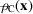be the electron-density map corresponding to the current atomic model, with structure factors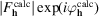; and let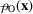be the map calculated from observed moduli and calculated phases, i.e. with coefficients. If there are enough data for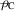to have a resolved peak at each model atomic position, then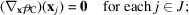while if the calculated phasesare good enough,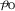will also have peaks at each: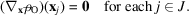It follows thatwhere the summation is over all reflections inor related toby space-group and Friedel symmetry (overlooking multiplicity factors!). This relation is less sensitive to series-termination errors than either of the previous two, since the spectrum ofcould have been extrapolated beyond the data inby using that of[as in van Reijen (1942)] without changing its right-hand side.

Cochran then used the identity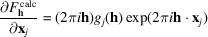in the form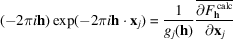to rewrite the previous relation as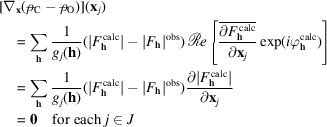(the operation[] on the first line being neutral because of Friedel symmetry). This is equivalent to the vanishing of thesubvector of the right-hand side of the normal equations associated to a least-squares refinement in which the weights would beCochran concluded that, for equal-atom structures withfor all j, the positionsobtained by Booth's method applied to the difference map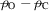are such that they minimize the residual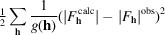with respect to the atomic positions. If it is desired to minimize the residual of the ordinary least-squares method, then the differential synthesis method should be applied to the weighted difference map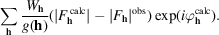He went on to show (Cochran, 1951b) that the refinement of temperature factors could also be carried out by inspecting appropriate derivatives of the weighted difference map.

This Fourier method was used by Freer et al. (1976)in conjunction with a stereochemical regularization procedure to refine protein structures.

References

Booth, A. D. (1948a). A new Fourier refinement technique. Nature (London), 161, 765–766.
Cochran, W. (1948b). The Fourier method of crystal structure analysis. Nature (London), 161, 765.
Cochran, W. (1948c). The Fourier method of crystal-structure analysis. Acta Cryst. 1, 138–142.
Cochran, W. (1951a). Some properties of the-synthesis. Acta Cryst. 4, 408–411.
Cochran, W. (1951b). The structures of pyrimidines and purines. V. The electron distribution in adenine hydrochloride. Acta Cryst. 4, 81–92.
Freer, S. T., Alden, R. A., Levens, S. A. & Kraut, J. (1976). Refinement of five protein structures by constrained Fo − Fc Fourier methods. In Crystallographic Computing Techniques, edited by F. R. Ahmed, pp. 317–321. Copenhagen: Munksgaard.
Reijen, L. L. van (1942). Diffraction effects in Fourier syntheses and their elimination in X-ray structure investigations. Physica, 9, 461–480.